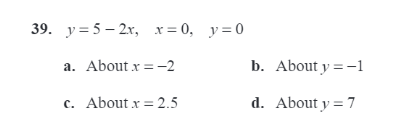# (Solved): Revolve The Region Bounded By The Graphs Of The Equation About The Given Line And Use Shell Method T...

Revolve the region bounded by the graphs of the equation about the given line and use shell method to find the volume of resulting solid.39. y = 5 - 2x, x = 0, y = 0 a. About x=-2 b. About y=-1 c. About x = 2.5 d. About y = 7

We have an Answer from Expert